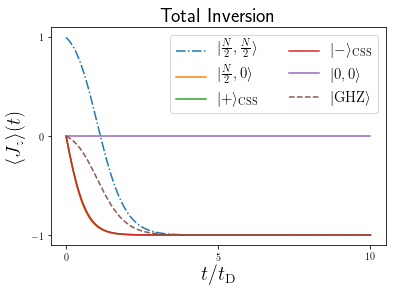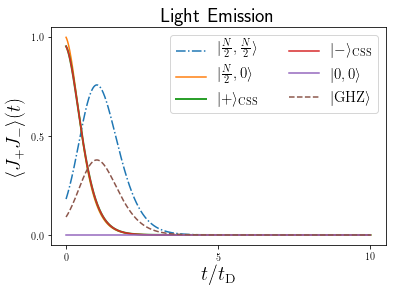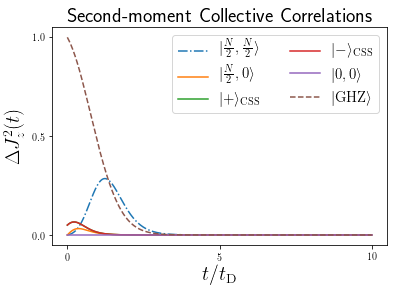Notebook author: Nathan Shammah (nathan.shammah at gmail.com)

We consider a system of $N$ two-level systems (TLSs) with identical frequency $\omega_{0}$, which can emit collectively at a rate $\gamma_\text{CE}$ , and suffer from dephasing and local losses at rates $\gamma_\text{D}$ and $\gamma_\text{E}$, respectively. The dynamics can be written as \begin{eqnarray} \dot{\rho} &=&-i\lbrack \omega_{0}J_z,\rho \rbrack +\frac{\gamma_\text {CE}}{2}\mathcal{L}_{J_{-}}[\rho] +\sum_{n=1}^{N}\frac{\gamma_\text{D}}{2}\mathcal{L}_{J_{z,n}}[\rho] +\frac{\gamma_\text{E}}{2}\mathcal{L}_{J_{-,n}}[\rho] \end{eqnarray}

When $\gamma_\text{E}=\gamma_\text{D}=0$ this dynamics is the classical superradiant master equation. In this limit, a system initially prepared in the fully-excited state undergoes superradiant light emission whose peak intensity scales proportionally to $N^2$.

This dynamics has been studied in Refs. [2-4] and implemented in various quantum optical platforms, including in the solid state [5-10]. A discussion of the difference between superradiant phase transition (typical of the Dicke model) and Dicke superradiance is present in Refs.  and .

Below, using PIQS  and QuTiP , we investigate the time evolution of the collective dynamics for an ensemble initialized in different initial quantum states.

Note that in the table above and in $\texttt{qutip.piqs}$ functions, the Lindbladian $\mathcal{L}[\rho]$ is written with a factor 1/2 with respect to $\mathcal{L}_{A}[\rho]$ reported in the LaTeX math equations, in order to have the Lindbladian and full Liouvillian matrix consistently defined by the rates $\gamma_\alpha$.

In :
from time import clock
import matplotlib.pyplot as plt
import scipy

from qutip import *
from qutip.piqs import *


In :
N = 20
ntls = N
nds = num_dicke_states(N)
print("The number of Dicke states is", nds)
[jx, jy, jz] = jspin(N)
jp = jspin(N, "+")
jm = jspin(N, "-")
system = Dicke(N = N)

The number of Dicke states is 121

In :
a = 1/np.sqrt(2)
b = 1/np.sqrt(2)
css_symmetric = css(N, a, b)
css_antisymmetric = css(N, a,-b)
excited_ = dicke(N, N/2,N/2)
ground_ = dicke(N,N/2,-N/2)
ghz_ = ghz(N)

In :
# here we set the initial coefficients
gE = 0 # local emission
gD = 0 # local dephasing
gP = 0 # local pumping
gCE = 1 # collective emission
gCD = 0 # collective dephasing
gCP = 0 # collective pumping
w0 = 1  # bare frequency
wi = 0  # coherent drive frequency

# spin hamiltonian
h0 = w0 * jz
hint = wi * jx
h = h0 #+ hint

#set initial conditions for spins by initializing the system and building the Liouvillian matrix
system = Dicke(hamiltonian = h,
N = N,
emission = gE,
pumping = gP,
dephasing = gD,
collective_emission = gCE,
collective_pumping = gCP,
collective_dephasing = gCD)
clock_t0 = clock()
liouv = system.liouvillian()
clock_tf = clock()
dt_clock = clock_tf - clock_t0
print("Time (in seconds) to generate the Liouvillian for N = 20 TLSs:", dt_clock)

Time (in seconds) to generate the Liouvillian for N = 20 TLSs: 0.05499199999999993

In :
## Solution of the dynamics for different initial conditions

# parameters for the time integration of the dynamics
nt = 1001
td0 = np.log(N)/(N*gCE) # delay time is used as a reference
tmax = 10 * td0
t = np.linspace(0, tmax, nt)

# initial states
rho01 = excited_
rho03 = css_symmetric
rho04 = css_antisymmetric
rho06 = ghz_

#Excited
clock_t0 = clock()
result1 = mesolve(liouv, rho01, t, [], e_ops = [jz, jp*jm, jz**2],
options = Options(store_states=True))
rhot1 = result1.states
jz_t1 = result1.expect
jpjm_t1 = result1.expect
jz2_t1 = result1.expect
clock_tf = clock()
dt_clock = clock_tf - clock_t0
print("Elapsed time (in seconds) for this run: ", dt_clock)

clock_t0 = clock()
result2 = mesolve(liouv, rho02, t, [], e_ops = [jz, jp*jm, jz**2],
options = Options(store_states=True))
rhot2 = result2.states
jz_t2 = result2.expect
jpjm_t2 = result2.expect
jz2_t2 = result2.expect
clock_tf = clock()
dt_clock = clock_tf - clock_t0
print("Elapsed time (in seconds) for this run: ", dt_clock)

#CSS Symmetric
clock_t0 = clock()
result3 = mesolve(liouv, rho03, t, [], e_ops = [jz, jp*jm, jz**2],
options = Options(store_states=True))
rhot3 = result3.states
jz_t3 = result3.expect
jpjm_t3 = result3.expect
jz2_t3 = result3.expect
clock_tf = clock()
dt_clock = clock_tf - clock_t0
print("Elapsed time (in seconds) for this run: ", dt_clock)

#CSS Antisymmetric
clock_t0 = clock()
result4 = mesolve(liouv, rho04, t, [], e_ops = [jz, jp*jm, jz**2],
options = Options(store_states=True))
rhot4 = result4.states
jz_t4 = result4.expect
jpjm_t4 = result4.expect
jz2_t4 = result4.expect
clock_tf = clock()
dt_clock = clock_tf - clock_t0
print("Elapsed time (in seconds) for this run: ", dt_clock)

clock_t0 = clock()
result5 = mesolve(liouv, rho05, t, [], e_ops = [jz, jp*jm, jz**2],
options = Options(store_states=True))
rhot5 = result5.states
jz_t5 = result5.expect
jpjm_t5 = result5.expect
jz2_t5 = result5.expect
clock_tf = clock()
dt_clock = clock_tf - clock_t0
print("Elapsed time (in seconds) for this run: ", dt_clock)

#GHZ
clock_t0 = clock()
result6 = mesolve(liouv, rho06, t, [], e_ops = [jz, jp*jm, jz**2],
options = Options(store_states=True))
rhot6 = result6.states
jz_t6 = result6.expect
jpjm_t6 = result6.expect
jz2_t6 = result6.expect
clock_tf = clock()
dt_clock = clock_tf - clock_t0
print("Elapsed time (in seconds) for this run: ", dt_clock)

Elapsed time (in seconds) for this run:  1.4128720000000001
Elapsed time (in seconds) for this run:  1.3064499999999994
Elapsed time (in seconds) for this run:  1.2567909999999998
Elapsed time (in seconds) for this run:  1.333939
Elapsed time (in seconds) for this run:  0.5167439999999992
Elapsed time (in seconds) for this run:  1.3920459999999997


#### Visualization¶

In :
jmax = (0.5 * N)
j2max = (0.5 * N + 1) * (0.5 * N)

label_size = 20
label_size2 = 20
label_size3 = 20
label_size4 = 15
plt.rc('text', usetex = True)

fig1 = plt.figure()
plt.plot(t/td0, jz_t1/jmax, '-.', label = r"$|\frac{N}{2},\frac{N}{2}\rangle$")
plt.plot(t/td0, jz_t2/jmax, '-', label = r"$|\frac{N}{2},0\rangle$")
plt.plot(t/td0, jz_t3/jmax, '-', label = r"$|+\rangle_\mathrm{CSS}$")
plt.plot(t/td0, jz_t4/jmax, '-', label = r"$|-\rangle_\mathrm{CSS}$")
plt.plot(t/td0,jz_t5/jmax, '-', label = r"$|0,0\rangle$")
plt.plot(t/td0,jz_t6/jmax, '--', label = r"$|\mathrm{GHZ}\rangle$")
#plt.ylim([-1,1])
plt.xlabel(r'$t/t_\mathrm{D}$', fontsize = label_size3)
plt.ylabel(r'$\langle J_{z} \rangle(t)$', fontsize = label_size3)
plt.xticks([0,(tmax/2)/td0,tmax/td0])
plt.yticks([-1,0,1])
plt.legend(fontsize = label_size4, ncol = 2)

# plot
plt.title(r'Total Inversion', fontsize = label_size3)
plt.show()
plt.close()

fig2 = plt.figure()
plt.plot(t/td0, jpjm_t1/j2max, '-.', label = r"$|\frac{N}{2},\frac{N}{2}\rangle$")
plt.plot(t/td0, jpjm_t2/j2max, '-', label = r"$|\frac{N}{2},0\rangle$")
plt.plot(t/td0, jpjm_t3/j2max, '-', label = r"$|+\rangle_\mathrm{CSS}$", linewidth = 2)
plt.plot(t/td0, jpjm_t4/j2max, '-', label = r"$|-\rangle_\mathrm{CSS}$")
plt.plot(t/td0,jpjm_t5/j2max, '-', label = r"$|0,0\rangle$")
plt.plot(t/td0,jpjm_t6/j2max, '--', label = r"$|\mathrm{GHZ}\rangle$")
#plt.ylim([0,1])
plt.xticks([0,(tmax/2)/td0,tmax/td0])
plt.yticks([0,0.5,1])
plt.xlabel(r'$t/t_\mathrm{D}$', fontsize = label_size3)
plt.ylabel(r'$\langle J_{+}J_{-}\rangle(t)$', fontsize = label_size3)
plt.legend(fontsize = label_size4, ncol = 2)

# plot
plt.title(r'Light Emission', fontsize = label_size3)
plt.show()
plt.close()

fig3 = plt.figure()
djz1 = (jz2_t1- jz_t1**2)/jmax**2
djz2 = (jz2_t2- jz_t2**2)/jmax**2
djz3 = (jz2_t3- jz_t3**2)/jmax**2
djz4 = (jz2_t4- jz_t4**2)/jmax**2
djz5 = (jz2_t5- jz_t5**2)/jmax**2
djz6 = (jz2_t6- jz_t6**2)/jmax**2

plt.plot(t/td0, djz1, '-.', label = r"$|\frac{N}{2},\frac{N}{2}\rangle$")
plt.plot(t/td0, djz2, '-', label = r"$|\frac{N}{2},0\rangle$")
plt.plot(t/td0, djz3, '-', label = r"$|+\rangle_\mathrm{CSS}$")
plt.plot(t/td0, djz4, '-', label = r"$|-\rangle_\mathrm{CSS}$")
plt.plot(t/td0, djz5, '-', label = r"$|0,0\rangle$")
plt.plot(t/td0, djz6, '--', label = r"$|\mathrm{GHZ}\rangle$")
#plt.ylim([-1,1])
plt.xticks([0,(tmax/2)/td0,tmax/td0])
plt.yticks([0,0.5,1])
plt.xlabel(r'$t/t_\mathrm{D}$', fontsize = label_size3)
plt.ylabel(r'$\Delta J_{z}^2(t)$', fontsize = label_size3)
plt.rc('xtick', labelsize=label_size)
plt.rc('ytick', labelsize=label_size)
plt.legend(fontsize = label_size4, ncol = 2)
plt.title(r'Second-moment Collective Correlations', fontsize = label_size3)
plt.show()
plt.close()We have found very different time evolutions for different quantum states that experience the same dynamics, leading to superradiant light emission, subradiance, delayed superradiant decay (superfluorescence), and macroscopic quantum correlations.

 R. H. Dicke, Coherence in spontaneous radiation processes, Phys. Rev. 93, 99 (1954)

 R. Bonifacio, P. Schwendimann, and F. Haake, Quantum statistical theory of superradiance. I, Phys. Rev. A 4, 302 (1971); ibid. 4, 854 (1971); R. Bonifacio and L. A. Lugiato, Cooperative radiation processes in two-level systems: Superfluorescence, Phys. Rev. A 11, 1507 (1975)

 N. Shammah, N. Lambert, F. Nori, and S. De Liberato, Superradiance with local phase-breaking effects, Phys. Rev. A 96, 023863 (2017)

 N. Shammah, S. Ahmed, N. Lambert, S. De Liberato, and F. Nori, Open quantum systems with local and collective incoherent processes: Efficient numerical simulation using permutational invariance, https://arxiv.org/abs/1805.05129 and https://github.com/nathanshammah/piqs/

 G. T. Noe II, J.-H. Kim, J. Lee, Y. Wang, A. K. Wojcik, S. A. McGill, D. H. Reitze, A. A. Belyanin, and J. Kono, Giant superfluorescent bursts from a semiconductor magneto-plasma, Nature Phys. 8, 219 (2012)

 T. Bienaimé, N. Piovella, and R. Kaiser, Controlled Dicke subradiance from a large cloud of two-level systems, Phys. Rev. Lett. 108, 123602 (2012)

 A. F. van Loo, A. Fedorov, K. Lalumière, B. C. Sanders, A. Blais, and A. Wallraff, Photon-mediated interactions between distant artificial atoms, Science 342, 1494 (2013)

 A. Goban, C.-L. Hung, J. D. Hood, S.-P. Yu, J. A. Muniz, O. Painter, and H. J. Kimble, Superradiance for atoms trapped along a photonic crystal waveguide, Phys. Rev. Lett. 115, 063601 (2015)

 C. Bradac, M. T. Johnsson, M. v. Breugel, B. Q. Baragiola, R. Martin, M. L. Juan, G. K. Brennen, and T. Volz, Room-temperature spontaneous superradiance from single diamond nanocrystals, Nature Commun. 8, 1205 (2017)

 A. Angerer, K. Streltsov, T. Astner, S. Putz, H. Sumiya, S. Onoda, W. J. Munro, K. Nemoto, J. Schmiedmayer, and J. Majer, Superradiant hybrid quantum devices, arXiv:1802.07100 (2018)

 P. Kirton and J. Keeling, Superradiant and lasing states in driven-dissipative Dicke models, New J. Phys. 20, 015009 (2018).

 J.R. Johansson, P.D. Nation, and F. Nori, Comp. Phys. Comm. 183, 1760 (2012) http://qutip.org

In :
qutip.about()

QuTiP: Quantum Toolbox in Python
A. J. Pitchford, P. D. Nation, R. J. Johansson, A. Grimsmo, and C. Granade

QuTiP Version:      4.3.1
Numpy Version:      1.14.2
Scipy Version:      1.1.0
Cython Version:     0.28.5
Matplotlib Version: 2.2.3
Python Version:     3.6.7
Number of CPUs:     2
BLAS Info:          INTEL MKL
OPENMP Installed:   False
INTEL MKL Ext:      True
Platform Info:      Darwin (x86_64)
Installation path:  /Users/nathanshammah/miniconda3/lib/python3.6/site-packages/qutip
==============================================================================
For your convenience a bibtex file can be easily generated using qutip.cite()